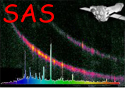XMM-Newton Science Analysis System

ommosaic (ommosaic-2.11) [xmmsas_20211130_0941-20.0.0]

## Image alignment

Two images are “aligned” by computing the cross-correlation coefficient R between them (please see section 11.5) for a range of x and y offset values that are added to the reference pixel coordinates of the second image. The x and y offsets that give the maximum R are subtracted from the reference pixel coordinates of the second image to align it with the first.

In the case of sky-images, the reference pixel coordinates is that given in the image file FITS header by the keywords CRPIX1 and CRPIX2.

In the case of unrotated images, the reference pixel coordinates is that given in the image file FITS header by the keywords WINDOWX0) and WINDOWY0.

The algorithm works as follows

```    Read the input parameter mincorr (the minimum correlation coefficient for a
successful correlation)

Take the absolute value of mincorr.
Find the size (n) of the vector of alignment paramters (see previous section)
Record that image i index of the value stored in alignment has been aligned
(this will be the reference image).

Loop from iter=1 to no upper bound
Loop through from k=1 to k=n
Obtain the image file indices i and j from aligment[k]
If neither image i or image j have been aligned skip this value of k
If both image i and image j have been aligned skip this value of k
If(image i has been aligned then cross-correlate image j with image i,
otherwise cross-correlate image i with image j
If the computed cross-correlation coefficient is at least equal to mincorr
then record that these two images have been aligned, store the correlation
coefficient and the x and y shifts between the two.
Otherwise record that these two images cannot be aligned.

End loop
If all the images have been aligned or none were aligned during the current
value of iter, exit the iter loop

End Loop
```

Subsections

XMM-Newton SOC -- 2021-11-30